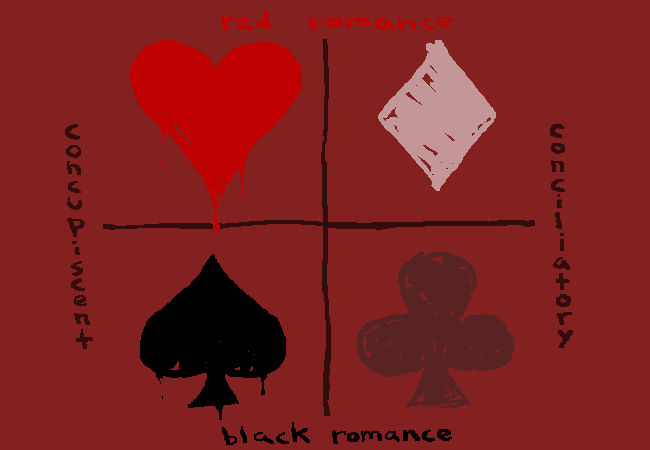# May Lord forgive us our sins.Wait, what about $\theta$ ?

Level pendingA) sine is algebraically less than the cosine for any angle between $2n\pi -\frac { 3\pi }{ 4 }$ and $2n\pi +\frac { \pi }{ 4 }$,where n is any integer.

×

Problem Loading...

Note Loading...

Set Loading...